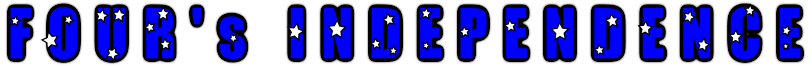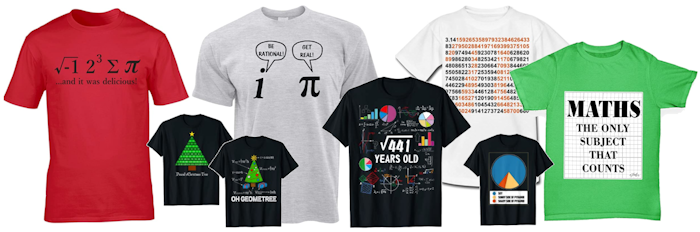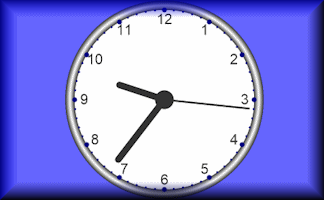#$$\frac{44}{44}$$
$$\frac{4\times4}{4+4}$$
$$\frac{4\times4-4}{4}$$
$$4+4\times(4-4)$$
$$\frac{4}{4}+\sqrt4+\sqrt4$$
$$\frac{4}{4}\times4+\sqrt4$$
$$\frac{44}{4}-4$$
$$4+4+4-4$$
$$4+4+\frac{4}{4}$$
$$\frac{44-4}{4}$$
$$\frac{44}{\sqrt4+\sqrt4}$$
$$\frac{44+4}{4}$$

A special clock for American Independence Day. It only uses the digit 4.
Can you design a special clock for a different day of the year using a different digit?

## A Mathematics Lesson Starter Of The Day

• Transum,
•
• An alternative way of using this Starter is to have it on the screen when the pupils are coming into the room then, after everyone has had chance to see it, turn off the projector and ask the class to reproduce it as best they can. Each number of the clock is replaced by four fours. Happy Independence day to all in the USA.
• Chistopher Boyle, St James's
•
• The clock got the four sign incorrect! It could have been 4 x (4/4).

[Transum: Thanks for your comment Christopher, but if you use the correct order of operations (BIDMAS) the multiplication should be done before the addition. The four is in fact correct. Your suggestion only includes 3 fours. Keep looking for errors though as feedback is very much appreciated]
•

How did you use this starter? Can you suggest how teachers could present or develop this resource? Do you have any comments? It is always useful to receive feedback and helps make this free resource even more useful for Maths teachers anywhere in the world.

Previous Day | This starter is for 4 July | Next Day

There are of course many ways pupils might respond to this challenge but here is an example of a solution

$$1=\left(\frac{9}{9}\right)^9$$

$$2=\left(\frac{9+9}{9}\right)$$

$$3=\sqrt{9}+9-9$$

$$4=\sqrt{9}+\frac{9}{9}$$

$$5=\sqrt{9}!-\frac{9}{9}$$

$$6=\sqrt{9}\times\sqrt{9}-\sqrt{9}$$

$$7=\sqrt{9}!+\frac{9}{9}$$

$$8=9-\frac{9}{9}$$

$$9=9+9-9$$

$$10=9+\frac{9}{9}$$

$$11=99\div9$$

$$12=9+\frac{9}{\sqrt{9}}$$

This type of challenge has been around for a long time. The first known reference is in a book called "The Schoolmasters Assistant: Being a Compendium of Arithmetic, Both Practical and Theoretical". It was written in 1762 by Thomas Dilworth, an English cleric. Here is the wording as it appeared in the book:$$33+\frac33 = 34$$

Your access to the majority of the Transum resources continues to be free but you can help support the continued growth of the website by doing your Amazon shopping using the links on this page. Below is an Amazon link. As an Amazon Associate I earn a small amount from qualifying purchases which helps pay for the upkeep of this website.

Educational Technology on Amazon

## GCSE Revision and Practice

Whatever exam board you use for GCSE Mathematics, this book by David Rayner remains an all-round winner. With this latest edition presented in full colour and completely updated for the new GCSE(9-1) specifications, this uniquely effective text continues to increase your chance of obtaining a good grade.

This book is targeted at the Higher tier GCSE, and provides a wealth of practice with careful progression, alongside substantial revision support for the new-style grading and exam questions. With all the new topics included, and a dedicated section on using and applying mathematics, this unique resource can be used either as a course book over two or three years or as a revision text in the run-up to exams. more...## Maths T-ShirtsMaths T-Shirts on AmazonTeacher, do your students have access to computers such as tablets, iPads or Laptops?  This page was really designed for projection on a whiteboard but if you really want the students to have access to it here is a concise URL for a version of this page without the comments: Transum.org/go/?Start=July4 However it would be better to assign one of the student interactive activities below.Here is the URL which will take them to a related student activity.

Transum.org/go/?to=onedigitHere is the link to the pairs game based on American-English and British-English mathematical words.

Transum.org/go/?to=MathvsMathsHere is a visual aid for teachers to use when teaching alalogue time.

Transum.org/go/?to=clockFor All: